# Mean State

Period Mean (original grids) [Watt m-2]
Model Period Mean (intersection) [Watt m-2]
Model Period Mean (complement) [Watt m-2]
Benchmark Period Mean (intersection) [Watt m-2]
Benchmark Period Mean (complement) [Watt m-2]
Bias [Watt m-2]
RMSE [Watt m-2]
Phase Shift [months]
Bias Score 
RMSE Score 
Seasonal Cycle Score 
Spatial Distribution Score 
Interannual Variability Score 
Overall Score 
Benchmark [-] 179.
CRUNCEPv7 [-] 192. 192. 0.00 177. 216. 14.5 24.4 1.07 0.43 0.45 0.86 0.93 0.51 0.61
GSWP3v1 [-] 183. 183. 0.00 177. 216. 5.93 16.8 0.514 0.62 0.52 0.94 0.92 0.73 0.71
WFDEI [-] 168. 167. 0.00 177. 216. -9.51 24.0 1.07 0.50 0.43 0.84 0.95 0.69 0.64
Period Mean (original grids) [Watt m-2]
Model Period Mean (intersection) [Watt m-2]
Model Period Mean (complement) [Watt m-2]
Benchmark Period Mean (intersection) [Watt m-2]
Benchmark Period Mean (complement) [Watt m-2]
Bias [Watt m-2]
RMSE [Watt m-2]
Phase Shift [months]
Bias Score 
RMSE Score 
Seasonal Cycle Score 
Spatial Distribution Score 
Interannual Variability Score 
Overall Score 
Benchmark [-] 189.
CRUNCEPv7 [-] 198. 198. 0.00 188. 224. 9.44 22.6 1.61 0.57 0.46 0.76 0.89 0.64 0.63
GSWP3v1 [-] 195. 195. 0.00 188. 224. 6.39 16.2 0.423 0.67 0.54 0.97 1.0 0.77 0.75
WFDEI [-] 190. 190. 0.00 188. 224. 2.13 19.5 0.534 0.61 0.50 0.94 1.0 0.74 0.72
Period Mean (original grids) [Watt m-2]
Model Period Mean (intersection) [Watt m-2]
Model Period Mean (complement) [Watt m-2]
Benchmark Period Mean (intersection) [Watt m-2]
Benchmark Period Mean (complement) [Watt m-2]
Bias [Watt m-2]
RMSE [Watt m-2]
Phase Shift [months]
Bias Score 
RMSE Score 
Seasonal Cycle Score 
Spatial Distribution Score 
Interannual Variability Score 
Overall Score 
Benchmark [-] 194.
CRUNCEPv7 [-] 195. 195. 0.00 192. 221. 2.95 22.4 1.07 0.69 0.50 0.86 0.73 0.53 0.64
GSWP3v1 [-] 197. 197. 0.00 192. 221. 4.44 18.6 0.496 0.71 0.57 0.93 0.96 0.67 0.73
WFDEI [-] 196. 196. 0.00 192. 221. 3.85 22.6 0.807 0.69 0.49 0.91 1.0 0.63 0.70
Period Mean (original grids) [Watt m-2]
Model Period Mean (intersection) [Watt m-2]
Model Period Mean (complement) [Watt m-2]
Benchmark Period Mean (intersection) [Watt m-2]
Benchmark Period Mean (complement) [Watt m-2]
Bias [Watt m-2]
RMSE [Watt m-2]
Phase Shift [months]
Bias Score 
RMSE Score 
Seasonal Cycle Score 
Spatial Distribution Score 
Interannual Variability Score 
Overall Score 
Benchmark [-] 81.3
CRUNCEPv7 [-] 72.0 72.1 0.00 80.9 86.2 -10.0 23.5 0.275 0.86 0.72 0.98 0.94 0.64 0.81
GSWP3v1 [-] 69.8 69.9 0.00 80.9 86.2 -11.6 21.8 0.200 0.84 0.74 0.99 0.94 0.72 0.83
WFDEI [-] 60.1 60.3 0.00 80.9 86.2 -21.0 29.0 0.271 0.74 0.71 0.98 0.92 0.79 0.81
Period Mean (original grids) [Watt m-2]
Model Period Mean (intersection) [Watt m-2]
Model Period Mean (complement) [Watt m-2]
Benchmark Period Mean (intersection) [Watt m-2]
Benchmark Period Mean (complement) [Watt m-2]
Bias [Watt m-2]
RMSE [Watt m-2]
Phase Shift [months]
Bias Score 
RMSE Score 
Seasonal Cycle Score 
Spatial Distribution Score 
Interannual Variability Score 
Overall Score 
Benchmark [-] 189.
CRUNCEPv7 [-] 187. 187. 0.00 183. 210. 6.23 26.2 1.53 0.48 0.41 0.77 0.55 0.47 0.52
GSWP3v1 [-] 178. 178. 0.00 183. 210. -1.23 20.6 0.686 0.60 0.45 0.91 0.73 0.71 0.64
WFDEI [-] 165. 165. 0.00 183. 210. -14.0 27.6 1.32 0.50 0.41 0.81 0.92 0.58 0.60
Period Mean (original grids) [Watt m-2]
Model Period Mean (intersection) [Watt m-2]
Model Period Mean (complement) [Watt m-2]
Benchmark Period Mean (intersection) [Watt m-2]
Benchmark Period Mean (complement) [Watt m-2]
Bias [Watt m-2]
RMSE [Watt m-2]
Phase Shift [months]
Bias Score 
RMSE Score 
Seasonal Cycle Score 
Spatial Distribution Score 
Interannual Variability Score 
Overall Score 
Benchmark [-] 105.
CRUNCEPv7 [-] 109. 109. 0.00 104. 127. 4.44 20.7 0.171 0.90 0.76 0.99 0.98 0.60 0.83
GSWP3v1 [-] 102. 102. 0.00 104. 127. -2.18 14.9 0.0819 0.95 0.81 0.99 1.0 0.63 0.87
WFDEI [-] 94.5 94.5 0.00 104. 127. -9.36 20.8 0.202 0.87 0.77 0.99 0.96 0.74 0.85
Period Mean (original grids) [Watt m-2]
Model Period Mean (intersection) [Watt m-2]
Model Period Mean (complement) [Watt m-2]
Benchmark Period Mean (intersection) [Watt m-2]
Benchmark Period Mean (complement) [Watt m-2]
Bias [Watt m-2]
RMSE [Watt m-2]
Phase Shift [months]
Bias Score 
RMSE Score 
Seasonal Cycle Score 
Spatial Distribution Score 
Interannual Variability Score 
Overall Score 
Benchmark [-] 140.
CRUNCEPv7 [-] 149. 149. 0.00 139. 154. 9.39 26.6 0.561 0.74 0.69 0.96 0.97 0.64 0.78
GSWP3v1 [-] 136. 136. 0.00 139. 154. -3.31 17.0 0.232 0.89 0.74 0.98 1.0 0.63 0.83
WFDEI [-] 142. 141. 0.00 139. 154. 1.92 24.5 0.553 0.83 0.66 0.95 0.96 0.72 0.80
Period Mean (original grids) [Watt m-2]
Model Period Mean (intersection) [Watt m-2]
Model Period Mean (complement) [Watt m-2]
Benchmark Period Mean (intersection) [Watt m-2]
Benchmark Period Mean (complement) [Watt m-2]
Bias [Watt m-2]
RMSE [Watt m-2]
Phase Shift [months]
Bias Score 
RMSE Score 
Seasonal Cycle Score 
Spatial Distribution Score 
Interannual Variability Score 
Overall Score 
Benchmark [-] 180.
CRUNCEPv7 [-] 195. 195. 0.00 178. 210. 16.4 31.7 0.424 0.53 0.54 0.97 0.96 0.53 0.68
GSWP3v1 [-] 175. 175. 0.00 178. 210. -1.06 18.7 0.290 0.80 0.62 0.98 0.93 0.64 0.76
WFDEI [-] 175. 175. 0.00 178. 210. -2.52 25.9 0.402 0.69 0.55 0.97 1.0 0.63 0.73
Period Mean (original grids) [Watt m-2]
Model Period Mean (intersection) [Watt m-2]
Model Period Mean (complement) [Watt m-2]
Benchmark Period Mean (intersection) [Watt m-2]
Benchmark Period Mean (complement) [Watt m-2]
Bias [Watt m-2]
RMSE [Watt m-2]
Phase Shift [months]
Bias Score 
RMSE Score 
Seasonal Cycle Score 
Spatial Distribution Score 
Interannual Variability Score 
Overall Score 
Benchmark [-] 74.6
CRUNCEPv7 [-] 63.7 64.1 0.00 74.6 74.8 -11.5 22.8 0.244 0.83 0.70 0.98 0.87 0.65 0.79
GSWP3v1 [-] 60.5 60.9 0.00 74.6 74.8 -14.4 20.3 0.228 0.80 0.74 0.98 0.91 0.68 0.81
WFDEI [-] 50.8 51.3 0.00 74.6 74.8 -24.0 28.6 0.237 0.69 0.71 0.98 0.94 0.76 0.80
Period Mean (original grids) [Watt m-2]
Model Period Mean (intersection) [Watt m-2]
Model Period Mean (complement) [Watt m-2]
Benchmark Period Mean (intersection) [Watt m-2]
Benchmark Period Mean (complement) [Watt m-2]
Bias [Watt m-2]
RMSE [Watt m-2]
Phase Shift [months]
Bias Score 
RMSE Score 
Seasonal Cycle Score 
Spatial Distribution Score 
Interannual Variability Score 
Overall Score 
Benchmark [-] 193.
CRUNCEPv7 [-] 193. 193. 0.00 193. 202. -1.42 20.6 0.505 0.83 0.68 0.97 0.94 0.58 0.78
GSWP3v1 [-] 190. 190. 0.00 193. 202. -3.35 18.2 0.157 0.85 0.71 0.99 1.0 0.61 0.81
WFDEI [-] 194. 194. 0.00 193. 202. -0.299 23.6 0.286 0.83 0.63 0.98 0.99 0.69 0.79
Period Mean (original grids) [Watt m-2]
Model Period Mean (intersection) [Watt m-2]
Model Period Mean (complement) [Watt m-2]
Benchmark Period Mean (intersection) [Watt m-2]
Benchmark Period Mean (complement) [Watt m-2]
Bias [Watt m-2]
RMSE [Watt m-2]
Phase Shift [months]
Bias Score 
RMSE Score 
Seasonal Cycle Score 
Spatial Distribution Score 
Interannual Variability Score 
Overall Score 
Benchmark [-] 120.
CRUNCEPv7 [-] 132. 132. 0.00 117. 151. 15.4 28.2 0.236 0.74 0.75 0.98 0.99 0.64 0.81
GSWP3v1 [-] 113. 113. 0.00 117. 151. -1.04 16.2 0.267 0.91 0.80 0.98 1.0 0.62 0.85
WFDEI [-] 113. 113. 0.00 117. 151. -1.91 23.0 0.292 0.87 0.73 0.98 0.97 0.74 0.84
Period Mean (original grids) [Watt m-2]
Model Period Mean (intersection) [Watt m-2]
Model Period Mean (complement) [Watt m-2]
Benchmark Period Mean (intersection) [Watt m-2]
Benchmark Period Mean (complement) [Watt m-2]
Bias [Watt m-2]
RMSE [Watt m-2]
Phase Shift [months]
Bias Score 
RMSE Score 
Seasonal Cycle Score 
Spatial Distribution Score 
Interannual Variability Score 
Overall Score 
Benchmark [-] 168.
CRUNCEPv7 [-] 143. 143. 0.00 143. 180. 0.159 24.0 0.587 0.71 0.63 0.94 0.95 0.55 0.74
GSWP3v1 [-] 137. 137. 0.00 143. 180. -5.71 18.9 0.261 0.79 0.67 0.97 0.97 0.62 0.78
WFDEI [-] 136. 136. 0.00 143. 180. -6.95 24.3 0.483 0.72 0.62 0.95 0.96 0.65 0.75
Period Mean (original grids) [Watt m-2]
Model Period Mean (intersection) [Watt m-2]
Model Period Mean (complement) [Watt m-2]
Benchmark Period Mean (intersection) [Watt m-2]
Benchmark Period Mean (complement) [Watt m-2]
Bias [Watt m-2]
RMSE [Watt m-2]
Phase Shift [months]
Bias Score 
RMSE Score 
Seasonal Cycle Score 
Spatial Distribution Score 
Interannual Variability Score 
Overall Score 
Benchmark [-] 175.
CRUNCEPv7 [-] 168. 168. 0.00 173. 224. -4.36 21.7 0.385 0.71 0.70 0.97 1.0 0.59 0.78
GSWP3v1 [-] 162. 162. 0.00 173. 224. -10.2 19.6 0.295 0.72 0.73 0.97 0.99 0.58 0.79
WFDEI [-] 172. 172. 0.00 173. 224. -0.873 20.7 0.465 0.74 0.69 0.96 0.99 0.56 0.77
Period Mean (original grids) [Watt m-2]
Model Period Mean (intersection) [Watt m-2]
Model Period Mean (complement) [Watt m-2]
Benchmark Period Mean (intersection) [Watt m-2]
Benchmark Period Mean (complement) [Watt m-2]
Bias [Watt m-2]
RMSE [Watt m-2]
Phase Shift [months]
Bias Score 
RMSE Score 
Seasonal Cycle Score 
Spatial Distribution Score 
Interannual Variability Score 
Overall Score 
Benchmark [-] 80.0
CRUNCEPv7 [-] 74.2 74.0 0.00 79.8 82.9 -7.97 22.3 0.115 0.86 0.73 0.99 0.81 0.63 0.79
GSWP3v1 [-] 69.1 69.0 0.00 79.8 82.9 -12.2 20.7 0.0842 0.83 0.75 0.99 0.91 0.74 0.83
WFDEI [-] 62.1 62.0 0.00 79.8 82.9 -19.3 28.1 0.176 0.75 0.71 0.99 0.85 0.77 0.80
Period Mean (original grids) [Watt m-2]
Model Period Mean (intersection) [Watt m-2]
Model Period Mean (complement) [Watt m-2]
Benchmark Period Mean (intersection) [Watt m-2]
Benchmark Period Mean (complement) [Watt m-2]
Bias [Watt m-2]
RMSE [Watt m-2]
Phase Shift [months]
Bias Score 
RMSE Score 
Seasonal Cycle Score 
Spatial Distribution Score 
Interannual Variability Score 
Overall Score 
Benchmark [-] 205.
CRUNCEPv7 [-] 189. 190. 0.00 200. 227. -8.91 28.6 0.818 0.61 0.59 0.92 0.66 0.52 0.65
GSWP3v1 [-] 192. 192. 0.00 200. 227. -6.02 20.0 0.221 0.77 0.64 0.98 0.90 0.62 0.76
WFDEI [-] 193. 194. 0.00 200. 227. -6.84 25.3 0.303 0.69 0.60 0.97 0.96 0.60 0.74
Period Mean (original grids) [Watt m-2]
Model Period Mean (intersection) [Watt m-2]
Model Period Mean (complement) [Watt m-2]
Benchmark Period Mean (intersection) [Watt m-2]
Benchmark Period Mean (complement) [Watt m-2]
Bias [Watt m-2]
RMSE [Watt m-2]
Phase Shift [months]
Bias Score 
RMSE Score 
Seasonal Cycle Score 
Spatial Distribution Score 
Interannual Variability Score 
Overall Score 
Benchmark [-] 153.
CRUNCEPv7 [-] 157. 157. 0.00 151. 194. 6.76 19.6 0.445 0.85 0.74 0.97 1.0 0.55 0.81
GSWP3v1 [-] 144. 144. 0.00 151. 194. -4.68 15.8 0.124 0.91 0.77 0.99 1.0 0.62 0.84
WFDEI [-] 150. 150. 0.00 151. 194. 0.713 22.8 0.281 0.91 0.68 0.98 0.99 0.75 0.83
Period Mean (original grids) [Watt m-2]
Model Period Mean (intersection) [Watt m-2]
Model Period Mean (complement) [Watt m-2]
Benchmark Period Mean (intersection) [Watt m-2]
Benchmark Period Mean (complement) [Watt m-2]
Bias [Watt m-2]
RMSE [Watt m-2]
Phase Shift [months]
Bias Score 
RMSE Score 
Seasonal Cycle Score 
Spatial Distribution Score 
Interannual Variability Score 
Overall Score 
Benchmark [-] 115.
CRUNCEPv7 [-] 123. 123. 0.00 114. 123. 8.11 20.5 0.210 0.87 0.78 0.99 0.97 0.58 0.83
GSWP3v1 [-] 112. 112. 0.00 114. 123. -2.84 14.6 0.0871 0.93 0.83 0.99 1.0 0.64 0.87
WFDEI [-] 111. 111. 0.00 114. 123. -3.11 19.9 0.148 0.91 0.77 0.99 0.94 0.79 0.86
Period Mean (original grids) [Watt m-2]
Model Period Mean (intersection) [Watt m-2]
Model Period Mean (complement) [Watt m-2]
Benchmark Period Mean (intersection) [Watt m-2]
Benchmark Period Mean (complement) [Watt m-2]
Bias [Watt m-2]
RMSE [Watt m-2]
Phase Shift [months]
Bias Score 
RMSE Score 
Seasonal Cycle Score 
Spatial Distribution Score 
Interannual Variability Score 
Overall Score 
Benchmark [-] 162.
CRUNCEPv7 [-] 156. 156. 0.00 162. 171. -5.22 17.5 0.262 0.90 0.79 0.98 0.99 0.61 0.84
GSWP3v1 [-] 160. 160. 0.00 162. 171. -1.80 14.7 0.134 0.93 0.81 0.99 1.0 0.65 0.87
WFDEI [-] 169. 169. 0.00 162. 171. 7.04 23.3 0.259 0.88 0.73 0.98 0.98 0.80 0.85
Period Mean (original grids) [Watt m-2]
Model Period Mean (intersection) [Watt m-2]
Model Period Mean (complement) [Watt m-2]
Benchmark Period Mean (intersection) [Watt m-2]
Benchmark Period Mean (complement) [Watt m-2]
Bias [Watt m-2]
RMSE [Watt m-2]
Phase Shift [months]
Bias Score 
RMSE Score 
Seasonal Cycle Score 
Spatial Distribution Score 
Interannual Variability Score 
Overall Score 
Benchmark [-] 176.
CRUNCEPv7 [-] 183. 183. 0.00 174. 226. 9.20 22.7 1.44 0.62 0.43 0.79 0.80 0.48 0.59
GSWP3v1 [-] 174. 174. 0.00 174. 226. 0.816 17.1 0.475 0.83 0.47 0.94 0.95 0.65 0.72
WFDEI [-] 160. 160. 0.00 174. 226. -14.4 26.8 1.44 0.49 0.40 0.81 1.0 0.53 0.61
Period Mean (original grids) [Watt m-2]
Model Period Mean (intersection) [Watt m-2]
Model Period Mean (complement) [Watt m-2]
Benchmark Period Mean (intersection) [Watt m-2]
Benchmark Period Mean (complement) [Watt m-2]
Bias [Watt m-2]
RMSE [Watt m-2]
Phase Shift [months]
Bias Score 
RMSE Score 
Seasonal Cycle Score 
Spatial Distribution Score 
Interannual Variability Score 
Overall Score 
Benchmark [-] 182.
CRUNCEPv7 [-] 185. 185. 0.00 181. 201. 3.85 23.1 0.998 0.79 0.53 0.88 0.91 0.47 0.68
GSWP3v1 [-] 181. 182. 0.00 181. 201. 0.0675 19.0 0.293 0.89 0.57 0.96 1.0 0.61 0.77
WFDEI [-] 175. 175. 0.00 181. 201. -6.25 24.9 0.569 0.77 0.51 0.94 1.0 0.68 0.73
Period Mean (original grids) [Watt m-2]
Model Period Mean (intersection) [Watt m-2]
Model Period Mean (complement) [Watt m-2]
Benchmark Period Mean (intersection) [Watt m-2]
Benchmark Period Mean (complement) [Watt m-2]
Bias [Watt m-2]
RMSE [Watt m-2]
Phase Shift [months]
Bias Score 
RMSE Score 
Seasonal Cycle Score 
Spatial Distribution Score 
Interannual Variability Score 
Overall Score 
Benchmark [-] 176.
CRUNCEPv7 [-] 177. 176. 0.00 176. 178. -1.34 28.1 0.536 0.72 0.66 0.95 0.82 0.51 0.72
GSWP3v1 [-] 178. 176. 0.00 176. 178. -2.72 20.8 0.309 0.84 0.70 0.97 1.0 0.60 0.80
WFDEI [-] 181. 181. 0.00 176. 178. 1.90 28.3 0.698 0.75 0.65 0.92 0.98 0.62 0.76

# Temporally integrated period mean

BENCHMARK MEAN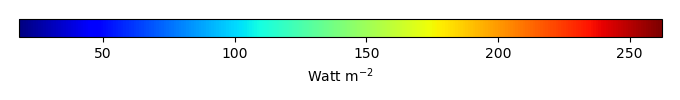MODEL MEANBIAS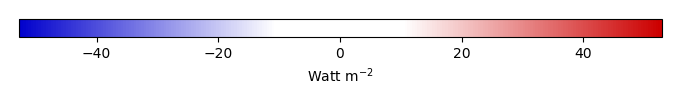BIAS SCORERMSE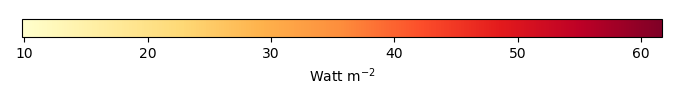RMSE SCOREBENCHMARK INTERANNUAL VARIABILITY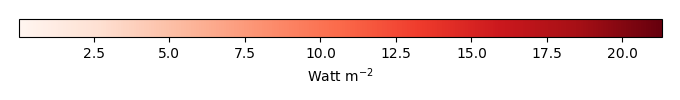MODEL INTERANNUAL VARIABILITYINTERANNUAL VARIABILITY SCOREBENCHMARK MAX MONTHMODEL MAX MONTHDIFFERENCE IN MAX MONTH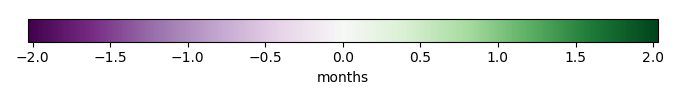SEASONAL CYCLE SCORESPATIAL TAYLOR DIAGRAMMODEL COLORS# Spatially integrated regional mean

MODEL COLORSREGIONAL MEANANNUAL CYCLEMONTHLY ANOMALYANNUAL CYCLE# All Models

BenchmarkCRUNCEPv7GSWP3v1WFDEI# Data Information

creation_date: Tue Jul 1 08:29:23 PDT 2014

source_file: This product is generated from monthly 1 degree GEWEX SRB Radiation observations